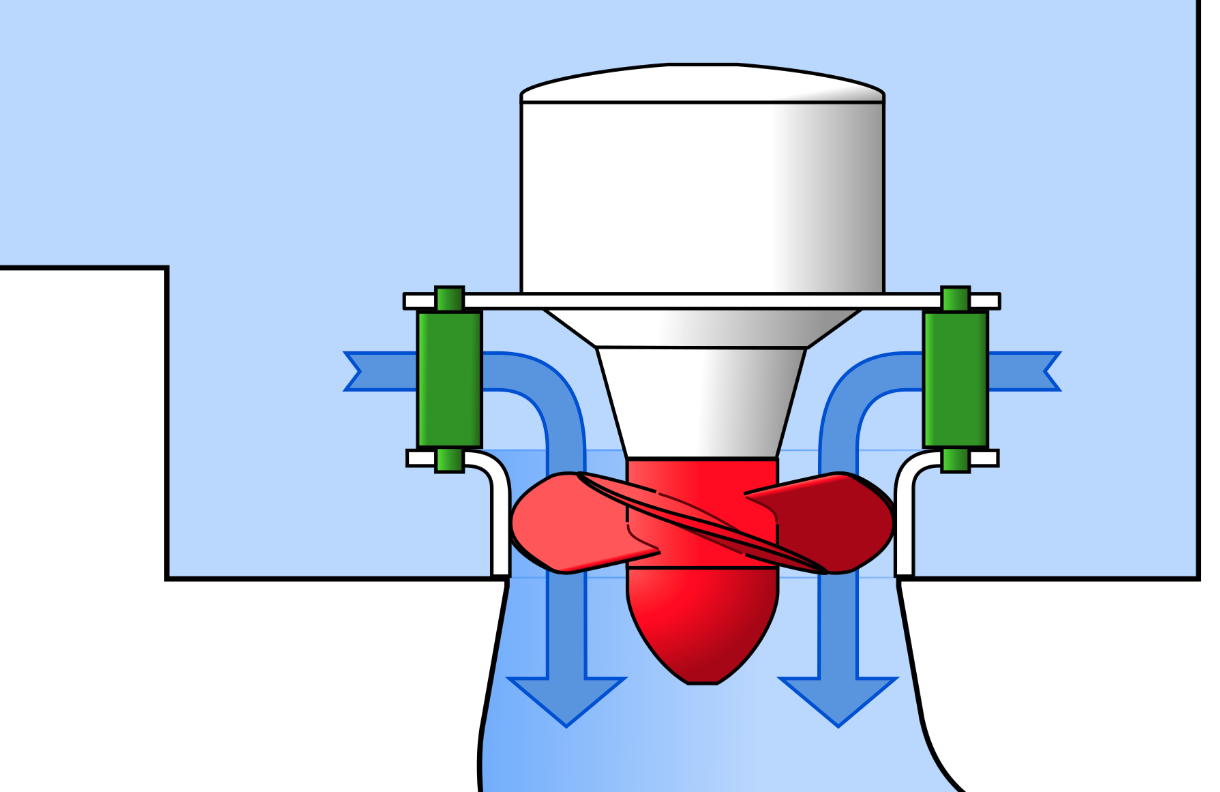# Optimal Rotational Speed of Kaplan and Francis Turbines (Technical Paper)Here's the first database in Francis and Kaplan turbines and my formula to estimate the optimal rotational speed!

Full title:  Optimal Rotational Speed of Kaplan and Francis Turbines with Focus on Low-Head Hydropower Applications and Dataset Collection

Author : Emanuele Quaranta, Ph.D.

Abstract : The rotational speed estimation of Francis and Kaplan turbines is of primary importance in the design of hydropower plants. However, most rotational speed equations were conceived for power outputs higher than 1 MW, i.e., for heads typically higher than 10 m for Kaplan turbines and 50 m for Francis turbines. Therefore, the accuracy of these equations is low when applied to reaction turbines with power outputs below 1 MW (mini-hydropower), which means the personal know-how of hydropower companies is generally used to select rotational speed. In light of this, a database composed of hundreds of full-scale Francis and Kaplan miniturbines was collected. Full-scale data were used in combination with the Buckingham π-theorem to determine a nondimensional equation for the rotational speed estimation of collected turbines. Flow rate and head were used as input variables. The dimensional analysis was also extended to high-head Kaplan and Francis turbines. A universal equation valid for both Francis and Kaplan turbines was obtained. Finally, the dimensionless terms obtained from the analysis were proposed as characteristic numbers for the selection of the hydraulic turbine type. The new equations were proposed as engineering tools for the design of hydroturbines.

Published in  Journal of Hydraulic Engineering, 2019

DOI : 10.1061/(ASCE)HY.1943-7900.0001643

Source: ASCE Library